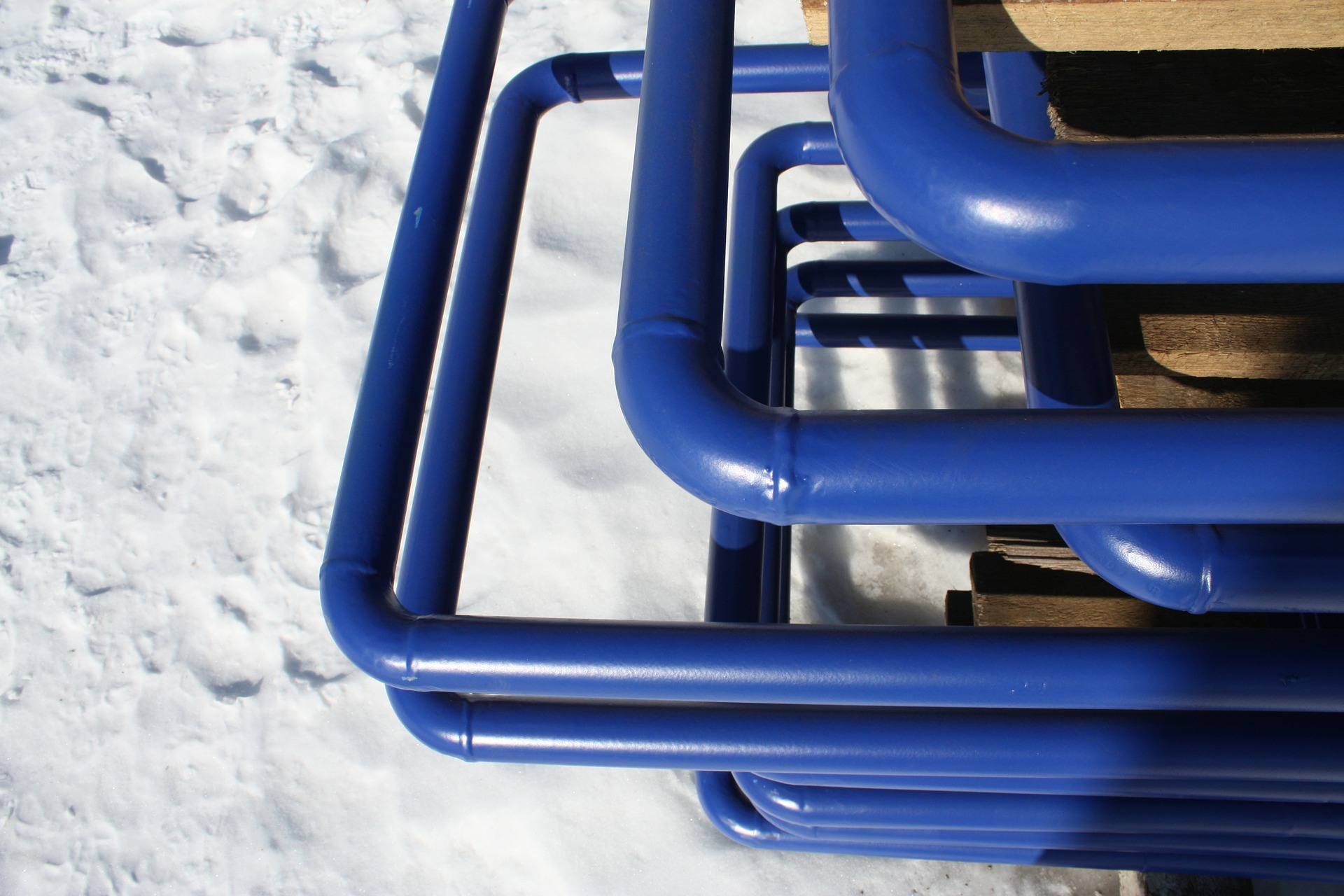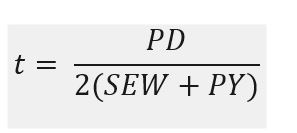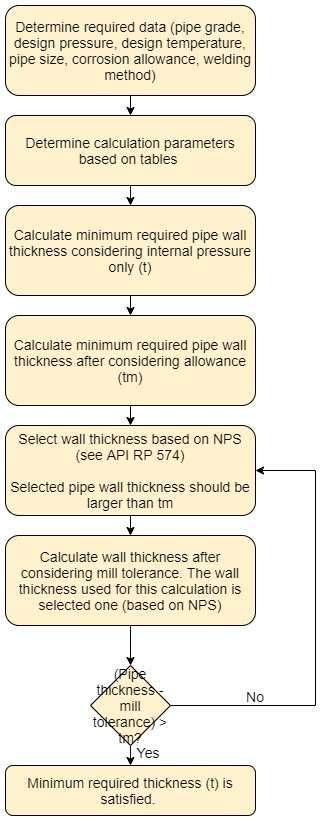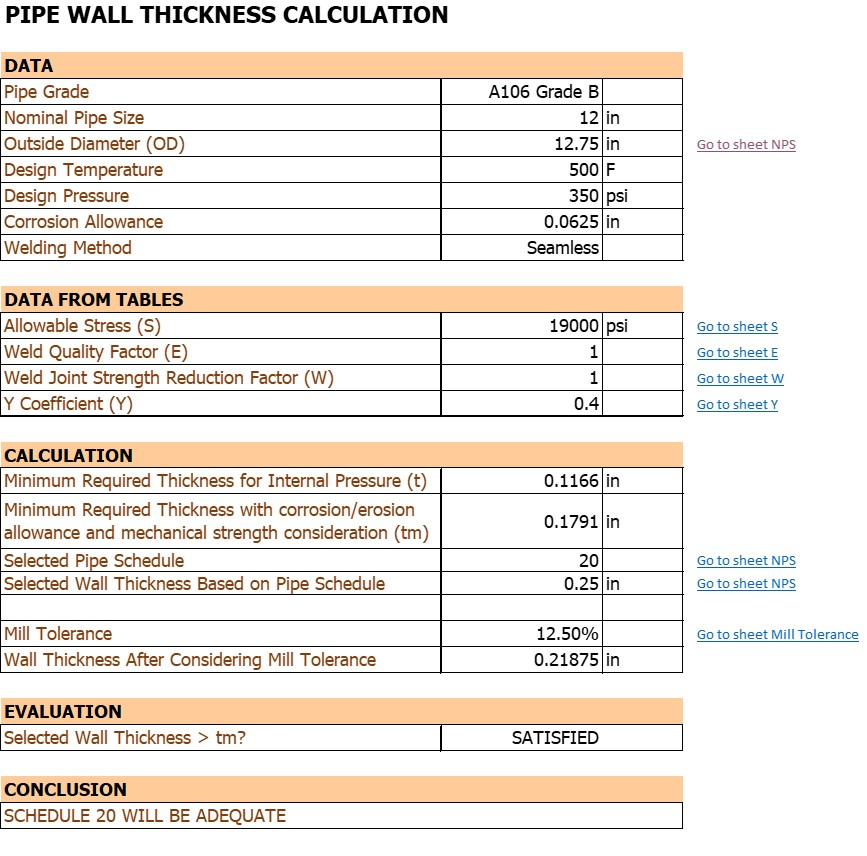# How To Calculate Required Wall Thickness and Schedule of Pipe

In this post I want to share how to calculate minimum required wall thickness and schedule of pipe. Before we jump into calculation steps, let’s review what pipe schedule is.

Pipe schedule expresses thickness of pipe. It is not actual measurement, but as a guide based on wall thickness formula.

Two pipes with the same diameter may have different schedules, which means they have a different wall thickness. So, somebody specifying a pipe for a high-pressure application will select a bigger number which represents a bigger schedule or thicker wall.

I made a simple spreadsheet on how to calculate required wall thickness and schedule of pipe. I found that the calculation is quite mechanical. Maybe some process engineers, even me, do not really used to this calculation. The calculation involves allowable stress, weld quality factor, etc. I do not think all process engineers should understand about how to calculate pipe wall thickness. But it would be better if we do.

## Required Data

To calculate pipe wall thickness, we need to have the following data:

• Nominal pipe size
• Design temperature
• Design pressure
• Corrosion allowance
• Welding method
• Mill tolerance

## Equations

Base on the data above, we will determine allowable stress (S), weld quality factor ( E ), weld joint strength reduction factor (W), and Y coefficient (Y). These parameters are used to calculate minimum required thickness for internal pressure only. The formula is:Where:

t              = minimum required thickness for internal pressure only

P             = design pressure (psi)

D            = outside diameter of pipe (in)

S             = allowable stress (psi)

E             = weld quality factor (unitless)

W           = weld joint reduction factor (unitless)

Y             = coefficient (unitless)

Minimum thickness of pipe must be at least equal to tm which considers allowance.

Where:

t              = minimum required thickness for internal pressure only

c             = the sum of mechanical allowances plus corrosion and erosion allowances (in)

tm           = minimum required wall thickness including allowances (in)

## Calculations Steps

Figure below shows step-by-step of pipe wall thickness calculation.

In these steps, the is “mill under tolerance” term. It is a value found in manufacturing specification of the particular pipe or plate from which the pipe is fabricated. For standard pipe, the mill under tolerance means that the pipe wall thickness could be 12.5% below the stated nominal thickness.